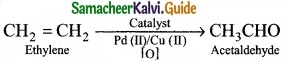Students can Download Tamil Nadu 11th Chemistry Model Question Paper 1 English Medium Pdf, Tamil Nadu 11th Chemistry Model Question Papers helps you to revise the complete Tamilnadu State Board New Syllabus, helps students complete homework assignments and to score high marks in board exams.

## TN State Board 11th Chemistry Model Question Paper 1 English Medium

General Instructions:

1. The question paper comprises of four parts.
2. You are to attempt all the parts. An internal choice of questions is provided wherever applicable.
3. All questions of Part I, II, III and IV are to be attempted separately.
4. Question numbers 1 to 15 in Part I are Multiple Choice Questions of one mark each.
These are to be answered by choosing the most suitable answer from the given four alternatives and writing the option code and the corresponding answer
5. Question numbers 16 to 24 in Part II are two-mark questions. These are to be answered in about one or two sentences.
6. Question numbers 25 to 33 in Part III are three-mark questions. These are to be answered in about three to five short sentences.
7. Question numbers 34 to 38 in Part IV are five-mark questions. These are to be answered in detail Draw diagrams wherever necessary.

Time: 2:30 Hours
Maximum Marks: 70

PART – I

Answer all the Questions: [15 × 1 = 15]
Choose the most suitable answer from the given four alternatives.

Question 1.
1 g of an impure sample of magnesium carbonate (containing no thermally decomposable impurities) on complete thermal decomposition gave 0.44 g of carbon dioxide gas. The percentage of impurity in the sample is …………………….
(a) 0%
(b) 4.4%
(c) 16%
(d) 8.4%
MgCO3 → MgO + CO2
MgCO3: (1 × 24) + (1 × 12) + (3 × 16) = 84g
CO2: (1 × 12) + (2 × 16) = 44g
100% pure 84 g MgCO3 on heating gives 44 g CO2
Given that 1 g of MgCO3 on heating gives 0.44 g CO2
Therefore, 84 g MgCO3 sample on heating gives 36.96 g CO2
Percentage of purity of the sample = $$\frac { 100% }{ 44gCO_{ 2 } }$$ × 36.96 g CO2 = 84%
Percentage of impurity = 16%
(c) 16%Question 2.
The electronic configuration of Eu (atomic no. 63) Gd (atomic no. 64) and Tb (atomic no. 65) are …………………….. [NEET – Phase II]
(a) [Xe] 4f6 5d1 6s2, [Xe] 4f7 5d1 6s2 and [Xe] 4f8 5d1 6s2
(b) [Xe] 4f7 6s2 [Xe] 4f8 6s2 and [Xe] 4f9 6s2
(c) [Xe] 4f7 6s2 [Xe] 4f7 5d1 6s2 and [Xe] 4f9 6s2
(d) [Xe] 4f 6 5d1 6s2, [Xe] 4f7 5d1 6s2 and [Xe] 4f9 6s2
Solution:
Eu : [Xe] 4f7, 5d0, 6s2
Gd : [Xe] 4f7, 5d1, 6s2
Tb : [Xe] 4f9, 5d0, 6s2
(b) [Xe] 4f7 6s2 [Xe] 4f8 6s2 and [Xe] 4f9 6s2Question 3.
Match the following: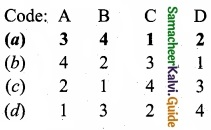Question 4.
Various successive ionization enthalpies (in kJ mol-1) of an element are given below.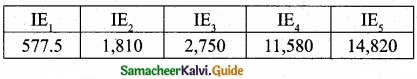The element is ……………………….
(a) Phosphorus
(b) Sodium
(c) Aluminium
(d) Silicon
(c) Aluminium

Question 5.
Non-stoichiometric hydrides are formed by ……………………..
(b) Carbon, nickel
(c) Manganese, lithium
(d) Nitrogen, chlorineQuestion 6.
Which is the function of sodium – potassium pump?
(a) Maintenance of ion balance
(b) Used in nerve impulse conduction
(c) Transmitting nerve signals
(d) Regulates the blood level
(c) Transmitting nerve signals

Question 7.
∆S is expected to be maximum for the reaction ………………………
(a) Ca(s) + 1/2O2(g) → CaO(s)
(b) C(s) + O2(g) → CO2(g)
(c) N2(g) + O2(g) → 2NO(g)
(d) CaCO3(s) → CaO(s) + CO2(g)
Solution:
In CaCO3(s) → CaO(s) + CO2(g) entropy change is positive. In (a) and (b) entropy change is negative; in (c) entropy change is zero.
(d) CaCO3(s) → CaO(s) + CO2(g)Question 8.
Which one of the following is a reversible reaction?
(a) Ripening of a banana
(b) Rusting of iron
(c) Tarnishing of silver
(d) Transport of oxygen by Hemoglobin in our body
Solution:
All the other three reactions are irreversible reactions. But the hemoglobin combines with O2 in lungs to form oxyhemoglobin. The oxyhemoglobin has a tendency to form hemoglobin by releasing O2. So it is a reversible reaction.
(d) Transport of oxygen by Hemoglobin in our body

Question 9.
P1 and P2 are the vapour pressures of pure liquid components, 1 and 2 respectively of an ideal binary solution if x1 represents the mole fraction of component 1, the total pressure of the solution formed by 1 and 2 will be ………………………….
Solution:
(a) P1 + x1 (P2 – P1)
(b) P2 – x1 (P2 + P1)
(c) P1 – x2 (P1 – P2)
(d) P1 + x2 (P1 – P2)
Ptotal = P1 + P2
= P1x1 + P2 x2
= P1(1 – x2) + P2x2 [∵x1 + x2 = 1, x1 = 1 – x2]
= P1 – P1x2 + P2x2 = P1 – x2 = P1 – x2 (P1 – P2)
(c) P1 – x2 (P1 – P2)Question 10.
Which of the following molecule contain no n bond?
(a) SO2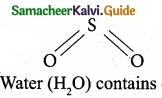(b) NO2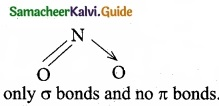(c) CO2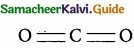(d) H2O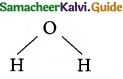Solution:
Water (H2O) contains only σ bonds and no π bonds.
(d) H2OQuestion 11.
IUPAC name ofis …………………….
(a) Trimethylheptane
(b) 2 -Ethyl -3, 3- dimethyl heptane
(c) 3, 4, 4 – Trimethyloctane
(d) 2 – Butyl -2 -methyl – 3 – ethyl-butane.
(c) 3, 4, 4 – Trimethyloctane

Question 12.
Which one of the following is an example for free radical initiators?
(a) Benzoyl peroxide
(b) Benzyl alcohol
(c) Benzyl acetate
(d) Benzaldehyde.
(a) Benzoyl peroxide

Question 13.
Some meta-directing substituents in aromatic substitution are given. Which one is most – deactivating?
(a) – COOH
(b) – NO2
(C) – C ≡ N
(d) – SO3H
(b) – NO2Question 14.
Consider the following statements.
(I) SN2 reaction is a bimolecular nucleophilic first order reaction.
(II) SN2 reaction take place in one step.
(III) SN2 reaction involves the formation of a carbocation. Which of the above statements is/are not correct?
(a) (II)
(b) (I) only
(c) (I) & (III)
(d) (III)
(c) (I) & (III)

Question 15.
The questions given below consists of an assertion and the reason. Choose the correct option out of the choices given below each question.
Assertion (A): If BOD level of water in a reservoir is more than 5 ppm it is highly polluted.
Reason(R): High biological oxygen demand means high activity of bacteria in water.
(a) Both (A) and R are correct and (R) is the correct explanation of (A)
(b) Both (A) and R are correct and (R) is not the correct explanation of (A)
(c) Both (A) and R are not correct
(d) (A) is correct but( R) is not correct
(d) (A) is correct but( R) is not correct

PART – II

Answer any six questions in which question No. 24 is compulsory. [6 × 2 = 12]

Question 16.
What is the actual configuration of copper (Z = 29)? Explain about its stability?
Copper (Z = 29)
Expected configuration : 1s2 2s2 2p6 3s2 3p6 3d9 4s2
Actual configuration : 1s2 2s2 2p6 3s2 3p6 3d10 4s1
The reason is that fully filled orbitals have been found to have extra stability.

Copper has the electronic configuration [Ar] 3d10 4s1 and not [Ar] 3d9 4s2 due the symmetrical distribution and exchange energies of d electrons.

Symmetry leads to stability. The full filled configuration have symmetrical distribution of electrons and hence they are more stable than unsymmetrical configuration.Question 17.
What is screening effect?
Screening effect:
The repulsive force between inner shell electrons and the valence electrons leads to a decrease in the electrostatic attractive forces acting on the valence electrons by the nucleus. Thus the inner shell elections act as a shield between the nucleus and the valence electrons. This effect is called shielding effect (or) screening effect.

Question 18.
Ice is less dense than water at 0°C. Justify this statement?
In ice, each oxygen atom is surrounded tetrahedrally by through hydrogen bonds to four water molecules. That is, the presence of two hydrogen atoms and two lone electron pairs (on oxygen) in each water molecule results in a three-dimensional structure. The arrangements creates an open structure, which in turn accounts for the fact that ice is less dense than water at 0°C.Question 19.
Aerosol cans carry clear warning of heating of the can. Why?
Aerosol cans carry clear warning of heating of the can. As the temperature rises, pressure in the can will increase and ambient temperatures about 120°F may lead to explosions.

So aerosol cans should always be stored in dry areas where they will not be exposed to excessive temperatures. You should never throw an aerosol can onto a fire, or leave it in the direct sunlight, even it is empty. This is because the pressure will build up so much that the can will burst. It is due to 2 reasons.

1. The gas pressure increases.
2. More of the liquefied propellant turns into a gas.

Question 20.
One mole of PCl5 is heated in one litre closed container. If 0.6 mole of chlorine is found at equilibrium, calculate the value of equilibrium constant?
Given that [PCl5]initial = $$\frac { 1mol }{ dm^{ 3 } }$$
[Cl2]eq = 0.6 mol dm-3
PCl5⇄ PCl3 + Cl2
[PCl5]eq = 0.6 mole dm-3
[PCl5]eq = 0.4 mole dm-3
∴ KC = $$\frac{0.6×0.6}{0.4}$$
KC = 0.9Question 21.
Why do gases always tend to be less soluble in liquids as the temperature is raised?
Mostly dissolution of gases in liquid is an exothermic process. It is because the fact that this process involves decrease of entropy.

Thus, increase of temperature tends to push the equilibrium towards backward direction as a result of which solubility of the gas decrease with rise in temperature.
(Gas + Solvent ⇄ Solution + Heat)

Question 22.
Give the general formula for the following classes of organic compounds?
(a) Aliphatic monohydric alcohol
(b) Aliphatic ketones
(c) Aliphatic amines.
(a) Aliphatic monohydric alcohol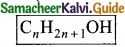(b) Aliphatic ketones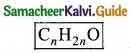(c) Aliphatic aminesQuestion 23.
Identify which of the following shows +1 and -I effect?

(I) -NO2
(II) -SO3H
(III) -I
(IV) -OH
(V) CH3O
(VI) CH3-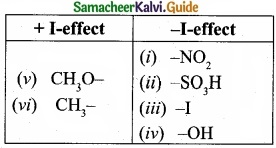Question 24.
Write the products A & B for the following reaction?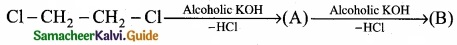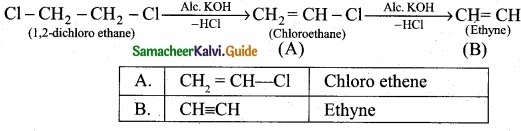PART – III

Answer any six questions in which question No. 33 is compulsory. [6 × 3 = 18]

Question 25.
Balance by oxidation number method: Mg + HNO3 → Mg(N03)2 + NO2 + H2O.
Step 1: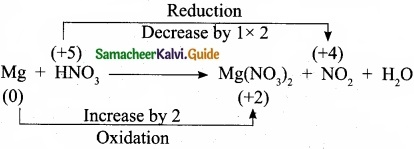Step 2:
Mg + 2HNO3 → Mg(NO3)2 + NG2 + H2O

Step 3:
To balance the number of oxygen atoms and hydrogen atoms 2HNO3 is multiplied by 2.
Mg + 4HNO3 → Mg(NO3)2 + 2NO2 + H2O

Step 4:
To balance the number of hydrogen atoms, the H2O molecule is multiplied by 2.
Mg + 4HNO3 → Mg(NO3)2 + 2NO2 + 2H2OQuestion 26.
For each of the following, give the sub level designation, the allowable m values and the number of orbitals?

1. n = 4, l = 2
2. n = 5, l = 3
3. n = 7, l = 0

1. n = 4, l = 2
If l = 2, ‘m’values are -2,-1, 0, +1, +2. So, 5 orbitals such as dxy, dyz ,dxz, $$d_{ x^{ 2 }-y^{ 2 } }$$ and $$d_{ z^{ 2 } }$$

2. n = 5, l = 3
If l = 3, ‘m’ values are -3, -2, -1, 0, +1, +2, +3
So, 7 orbitals such as $$\mathrm{f}_{\mathrm{z}}^{3}, \mathrm{f}_{\mathrm{xz}}^{2}, \mathrm{f}_{\mathrm{yz}}^{2}, \mathrm{f}_{\mathrm{xyz}}, \mathrm{f}_{\mathrm{z}\left(\mathrm{x}^{2}-\mathrm{y}^{2}\right)}, \mathrm{f}_{\mathrm{x}\left(\mathrm{x}^{2}-3 \mathrm{y}^{2}\right)}, \mathrm{f}_{\mathrm{y}\left(3 \mathrm{x}^{2}-\mathrm{z}^{2}\right)}$$

3. n = 7, l = 0
If l = 0, ‘m’ values are 0. Only one value. So, 1 orbital such as 7s orbital.Question 27.
Prove that ionization energy is a periodic property?
Variation in a period:
On moving across a period from left to right, the ionization enthalpy value increases.
This is due to the following reasons:

1. Increase of nuclear charge in a period
2. Decrease of atomic size in a period

Because of these reasons, the valence electrons are held more tightly by the nucleus, thus ionization enthalpy increases. Hence, ionization energy is a periodic property.

Variation in a group:
As we move from top to bottom along a group, the ionization enthalpy decreases. This is due to the following reasons:

1. A gradual increase in atomic size
2. Increase of screening effect on the outermost electrons due. to the increase of number of inner electrons.

Hence, ionization enthalpy is a periodic property.Question 28.
Explain how heat absorbed at constant pressure is measured using coffee cup calorimeter with neat diagram?

1. Measurement of heat change at constant pressure can be done in a coffee cup calorimeter.
2. We know that ∆H = qp (at constant P) and therefore, heat absorbed or evolved, qp at constant pressure is also called the heat of reaction or enthalpy of reaction, ∆Hr
3. In an exothermic reaction, heat is evolved, and system loses heat to the surroundings. Therefore, qp will be negative and ∆Hr will Reaction also be negative. Mixture
4. Similarly in an endothermic reaction, heat is absorbed, qp is positive and ∆Hr will also be positive.Question 29.
Consider the following reactions,
(a) H2(g) + I2(g) ⇄ 2 HI(g)
(b) CaCO2(s) ⇄ CaO(s) + CO2(g)
(c) S(s) + 3F2(g) ⇄ SF6(g)
In each of the above reaction find out whether you have to increase (or) decrease the volume to increase the yield of the product?
(a) H2(g) + I22(g) ⇄ 2HI(g)
In the above equilibrium reaction, volume of gaseous mdlecules is equal on both sides. So increase or decrease the volume will not affect the equilibrium and there will be no change in the yield of product.

(b)Volume is greater in product side. By decreasing the pressure, volume will increase thus, to get more of product CO2, the pressure should be decreased or volume should be increased.

(c)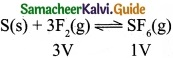Volume is lesser in product side. So by increasing the pressure, equilibrium shifts to the product side.Question 30.
You are provided with a solid ‘A’ and three solutions of A dissolved in water one saturated, one unsaturated, and one super saturated. How would you determine each solution?
(I) Saturated solution:
When maximum amount of solute is dissolved in a solvent, any more addition of solute will result in precipitation at a given temperature and pressure. Such a solution is called a saturated solution.

(II) Unsaturated solution:
When minimum amount of solute is dissolved in a solvent at a given temperature and pressure is called an unsaturated solution.

(III) Super saturated solution:
It is a solution that holds more solute than it normally could in its saturated form.

Example:

1. A saturated solution where the addition of more compound would not dissolve in the solution. 359 g of NaCl in 1 litre of water at 25°C.
2. An unsaturated solution has the capacity to dissolve more of the compound. 36 g of NaCl in 1 litre of water at 25°C.
3. A super saturated solution is the solution in which crystals can start growing. 500 g of NaCl in 1 litre of water at 25°C.Question 31.
Explain about the salient features of molecular orbital theory?

• When atoms combine to fonn molecules, their individual atomic orbitals lose their identity and form new orbitals called molecular orbitals.
• The shape of molecular orbitals depend upon the shapes of combining atomic orbitals.
• The number of molecular orbitals formed is the same as the number of combining atomic orbitals. Half the number of molecular orbitals formed will have lower energy and are called bonding orbitals, while the remaining half molecular orbitals will have higher energy and are called anti-bonding molecular orbitals.
• The bonding molecular orbitals are represented as σ (sigma), π (pi), δ (delta) and the corresponding anti-bonding orbitals are called C*, 7t* and 5*.
• The electrons in the molecule are accommodated in the newly formed molecular orbitals. The filling of electrons in these orbitals follow Aufbau’s Principle, Pauli’s exclusion principle and Hund’s rule as in the case of filling of electrons in the atomic orbitals.
• Bond order gives the number of covalent bonds between the two combining atoms.

The bond order of a molecule can be calculated using the following equation:
Bond order = $$\frac { N_{ b }-N_{ a } }{ 2 }$$
Nb = Number of electrons in bonding molecular orbitals.
Na = Number of electrons in anti-bonding molecular orbitals.
(vii) A bond order of zero value indicates that the molecule does not exist.Question 32.
Explain the types of addition reactions?
Addition reactions are classified into three types they are,

1. Electrophilic addition reaction
2. Nucleophilic addition reaction

1. Electrophilic addition reaction:
An electrophilic addition reaction can be described as an addition reaction in whifth a reactant with multiple bonds as in a double or triple bond undergoes has its n bond broken and two new a bond are formed.(ethane) Br Bf

2. Nucleophilic addition reaction:
A nucleophilic addition reaction is an addition reaction where a chemical compound with an electron deficient or electrophilic double or triple bond, a n bond, reacts with a nucleophilic which is an electron rich reactant with the disappearance of the double bond and creation of two new single or a bonds.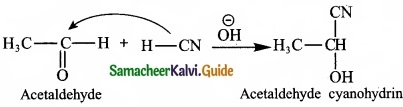It is an addition reaction in organic chemistry involving free radicals. The addition may occur between a radical and a non radical or between two radicals.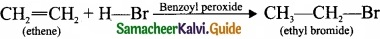Question 33.
Complete the following reaction and identify A, B and C?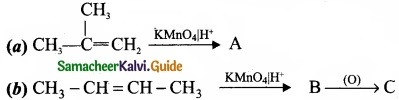PART – IV

Answer all the questions. [5 × 5 = 25]

Question 34 (a).
(I) How many radial nodes for 2s, 4p, 5d and 4f orbitals exhibit? How many angular nodes?
(II) How many unpaired electrons are present in the ground state of

(a) Cr3+ (Z = 24)
(b) Ne (Z = 10)

[OR]

(b) (I) The electronic configuration of an atom is one of the important factor which affects the value of ionization potential and electron gain enthalpy. Explain?
(II) Explain why cation are smaller and anions are larger in radii than their parent atoms?
(a) (I) Formula for total number of nodes = n – 1
For 2s orbital: Number of radial nodes = 1.
For 4p orbital: Number of radial nodes = n – l – 1.
= 4 – 1 – 1 = 2
Number of angular nodes = l
∴Number of angular nodes = l.
So, 4p orbital has 2 radial nodes and 1 angular node.

For 5d orbital:
Total number of nodes = n – 1
= 5 – 1 =4 nodes
Number of radial nodes = n – l – 1
= 5 – 2 – 1 = 2 radial nodes.
Number of angular nodes = l = 2
∴5d orbital have 2 radial nodes and 2 angular nodes.

For 4f orbital:
Total number of nodes = n – 1
= 4 – 1 = 3 nodes
Number of radial nodes = n – l – 1
= 4 – 3 – 1 = 0 node.
Number of angular nodes = l
= 3 nodes
∴ 5d orbital have 0 radial node and 3 angular nodes.

(II) (a) Cr (Z = 24) 1s2 2s2 2p6 3s2 3p6 3d5 4s1
Cr3+ – 1s2 2s2 2p6 3s2 3p6 3d4.
It contains 4 unpaired electrons.
(b) Ne(Z=10) 1s2 2s2 2p6. No unpaired electrons in it.

[OR]

(b) (I)

• Electronic configuration of an atom affects the value of ionization potential and electron gain enthalpy.
• Half filled valence shell electronic configuration and completely filled valence shell electronic configuration are more stable than partially filled electronic configuration.
• For e.g. Beryllium (Z = 4) 1s2 2s2 (completely filled electronic configuration)
Nitrogen (Z = 7) 1s2 2s2 2px1 2py1 2pz1 (half filled electronic configuration)
Both beryllium and nitrogen have high ionization energy due to more stable nature.
• In the case of beryllium (1s2 2s2), nitrogen (1s2 2s2 2p3) the addition of extra electron will disturb their stable electronic configuration and they have almost zero electron affinity.
• Noble gases have stable ns2 np6 configuration and the addition of further electron is unfavorable and they have zero electron affinity.

(II) A cation is smaller than the parent atom because it has fewer electrons while its nuclear charge remains the same. The size of anion will be larger than that of parent atom because the addition of one or more electrons would result in increased repulsion among the electrons and a decrease in effective nuclear charge.Question 35 (a).
(I) Arrange NH3, H2O and HF in the order of increasing magnitude of hydrogen bonding and explain the basis for your arrangement?
(II) Can we use concentrated sulphuric acid and pure zinc in the preparation of dihydrogen?

[OR]

(b) (I) Beryllium halides are covalent whereas magnesium halides are ionic. Why?
(II) What happens when
Sodium metal is dropped in water?

1. Sodium metal is heated in free supply of air?
2. Sodium peroxide dissolves in water?

(a) 1. Increasing magnitude of hydrogen bonding among NH3, H2O and HF is
HF > H2O > NH3

2. The extent of hydrogen bonding depends upon electronegativity and the number of hydrogne atoms available for bonding.

3. Among N, F and O the increasing order of their electronegativities are
N < O < F 4. Hence the expected order of the extent of hydrogen bonding is HF > H2O > NH3

(II) Conc. H2SO4 cannot be used because it acts as an oxidizing agent also and gets reduced to SO2.
Zn + 2H2SO4 (Conc) → ZnSO4 + 2H2O + SO2 Pure Zn is not used because it is non-porous and reaction will be slow. The impurities in Zn help in constitute of electrochemical couple and speed up reaction.

[OR]

(b)
(I) Beryllium ion (Be2+) is smaller in size and it is involved in equal sharing of electrons with halogens to form covalent bond, whereas magnesium ion (Mg2+) is bigger and it is involved in transfer of electrons to form ionic bond.

(II)

1. 2Na + 2H2O → 2NaOH + H2
2. 2Na + O2 → Na2O2
3. Na2O2 + 2H2O → 2NaOH + H2O2Question 36 (a).
(I) Define Gibb’s free energy?
(II) You are given normal boiling points and standard enthalpies of vapourisation. Calculate the entropy of vapourisation of liquids listed below?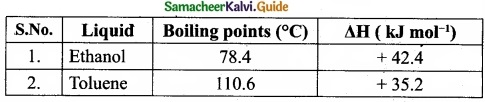[OR]

(b) (I) Define mole fraction.
(II) Differentiate between ideal solution and non-ideal solution.
(a) (I) Gibbs free energy is defined as the part of total energy of a system that can be converted (or) available for conversion into work.
G = H – TS, where G = Gibb’s free energy
H – enthalpy;
T = temperature;
S = entropy

(II) For ethanol:
Given: Tb = 78.4°C = (78.4 + 273) = 351.4 K[OR]

(b) (I) Mole fraction of a component is the ratio of number of moles of the component to the total number of moles of all components present in the solution.(II)
Ideal Solution:

1. An ideal solution is a solution in which each component obeys the Raoult’s law over entire range of concentration.
2. For an ideal solution,
ΔHmissing = 0, ΔVmixing = 0
3. Example: Benzene and toulene n – Hexane and n – Heptane.

Non – Ideal Solution:

1. The solution which do not obey Raults’s law over entire range of concentrations are called non-ideal solution.
2. For an ideal solution,
ΔHmixing ≠ 0, ΔVmixing ≠ 0
3. Example: Ethyl alcohol and Cyclo hexane, Benzene and aceton.Question 37 (a).
(I) What is dipole moment?
(II) Describe Fajan’s rule?

[OR]

(b) (I) How does Huckel rule help to decide the aromatic character of a compound?
(II) Draw cis-trans isomers for the following compounds
(a) 2- chloro-2-butene
(b) CH3-CCl=CH-CH2CH3
(a)
(I)

1. The polarity of a covalent bond can be measured in terms of dipole moment which is defined as: m = q × 2d, where m is the dipole moment, q is the charge, 2d is the distance between the two charges.
2. The dipole moment is a vector quantity and the direction of the dipole moment points from the negative charge to positive charge.
3. The unit of dipole moment is Coulomb metre (C m). It is usually expressed in Debye unit (D).
4. 1 Debye = 3.336 × 10-3o C m

(II)

1. The ability of a cation to polarise an anion is called its polarising ability and the tendency of ah anion to get polarised is called its polarisibility. The extent of polarisation in an ionic compound is given by the Fajans rule.

2. To show greater covalent character, both the cation and anion should have high charge on them. Higher the positive charge on the cation greater will be the attraction on the electron cloud of the anion. Similarly higher the magnitude of negative charge on anion, greater is its polarisability. For example, Na+ < Mg2+ < Al3+, the covalent character also follows the order:
NaCl < MgCl2 < AlCl3.

3. The smaller cation and larger anion show greater covalent character due to the greater extent of polarisation, e.g., LiCl is more covalent than NaCl.

4. Cation having ns2 np6 nd0 configuration shows greater polarising power than the cations with ns2 np6 configuration, e.g., CuCl is more covalent than NaCl.

[OR]

(b) (I) A compound is said to be aromatic, if it obeys the following rules:

1. The molecule must be cyclic.
2. The molecule must be co-planar.
3. Complete delocalisation of rc-electrons in the ring.
4. Presence of (4n + 2)π electrons in the ring where n is an integer (n = 0, 1, 2 …)

This is known as Huckel’s rule.
Example –– Benzene

1. It is cyclic one.
2. It is a co-planar molecule.
3. It has six delocalised n electrons.
4. 4n + 2 = 6

4n = 6 – 2
4n = 4
⇒ n = 1
It obey Huckel’s rule, with n = 1, hence benzene is aromatic in nature.

(II) (a) 2-Chloro-2-butene:

(b)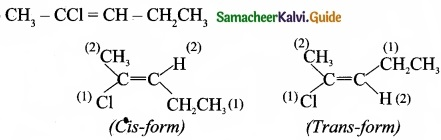Question 38 (a).
(I) Reagents and the conditions used in the reactions are given below. Complete the table by writing down the product and the name of the reaction?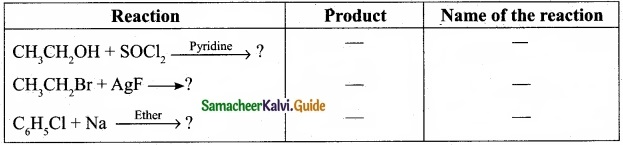(II) What is the IUPAC name of the insecticide DDT? Why is their use banned in most of the countries?

[OR]

(b) (I) Explain about green chemistry in day-to-day life?
(II) How acetaldehyde is commercially prepared by green chemistry?
(a)
(I)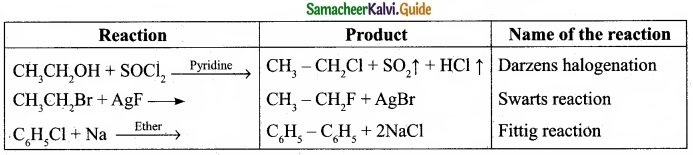(II)

•  The IUPAC name of the insecticide DDT is p, p’-dichloro-diphenyl trichloroethane.
• Even DDT is an effective insecticide. Now-adays it is banned because of its long term toxic effects.
• DDT is very persistent in the environment and it has a high affinity for fatty tissues. As a result, DDT gets accumulated in animal tissue fat, in particular that of birds of prey with subsequent thinning of their eggs shells and impacting their rate of reproduction. That is why DDT is banned in most of the countries.

[OR]

(b)
(I)
1. Dry cleaning of clothes:
Solvents like tetrachloroethylene used in dry cleaning of clothes, pollute the ground water and are carcinogenic. In place of tetrachloro ethylene, liquefied CO2 with suitable detergent is an alternate solvent used. Liquefied CO2 is not harmful to the ground water. Nowadays H2O2 is used for bleaching clothes in laundry, gives better result and utilises less water.

2. Bleaching of paper:
Conventional method of bleaching was done with chlorine. Nowadays H2O2 can be used for bleaching paper in the presence of catalyst.

1. Instead of petrol, methaftol is used as a fuel in automobiles.
2. Neem based pesticides have been synthesised, which are more safer than the chlorinated hydrocarbons.

(II) Acetaldehyde is commericially prepared by one step oxidation of ethene in the presence of ionic catalyst in aqueous medium with 90% yield.